## How to use the calculator function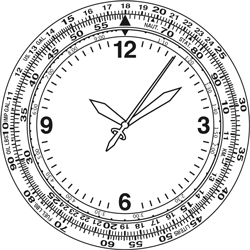Note: In some models, the inner and outer scales are opposite. Be sure to make the appropriate substitutions to the following instructions.

## Simple Calculations

### [How to do multiplication]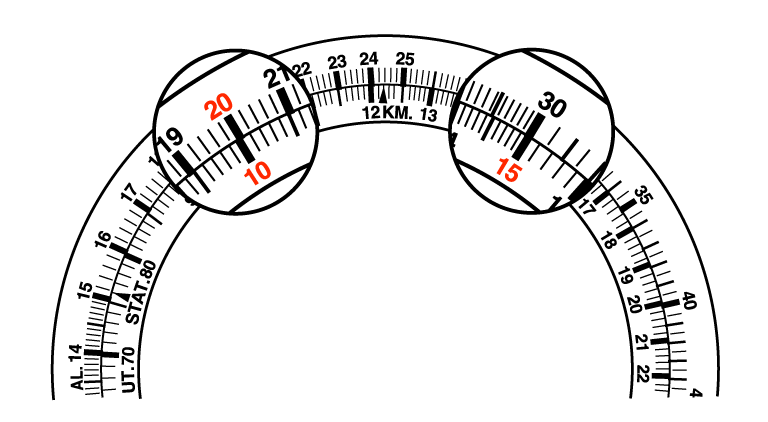Q :
20 × 15

A :
Adjust 20 of the outer scale until it points to 10 of the inner scale.
You can read the digit 30 from of the scale of the outer scale corresponding to 15 of the inner scale and adding a unit, you can get the answer 300.

### [How to do division]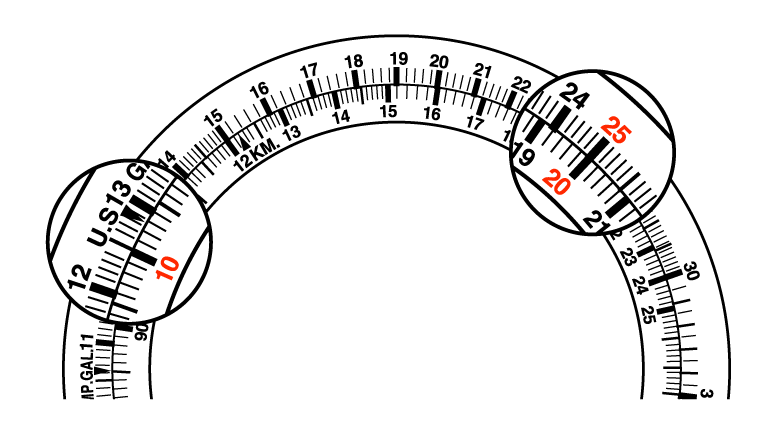Q :
250 ÷ 20

A :
Adjust 25 of the outer scale until it points to 20 of the inner scale.
You can read the digit 12.5 from of the scale of the outer scale corresponding to 10 of the inner scale and get the answer 12.5.

### [How to calculate ratios]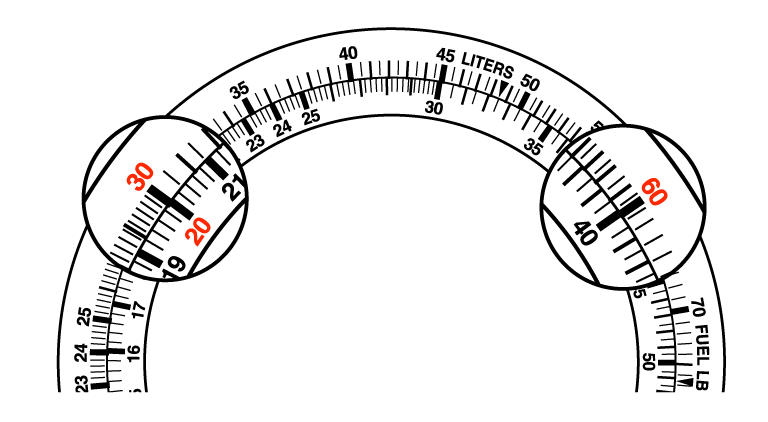Q :
30/20 = 60/A

A :
Adjust 30 of the outer scale until it points to 20 of the inner scale.
You can read the digit 40 from of the scale of the inner scale corresponding to 60 of the outer scale and on all the positions above the scale, the ratio between “inner and outer” is the ratio between “30 and 20”. Therefore, you can determine the answers of other ratios.

### [How to convert volume between different units of measurement]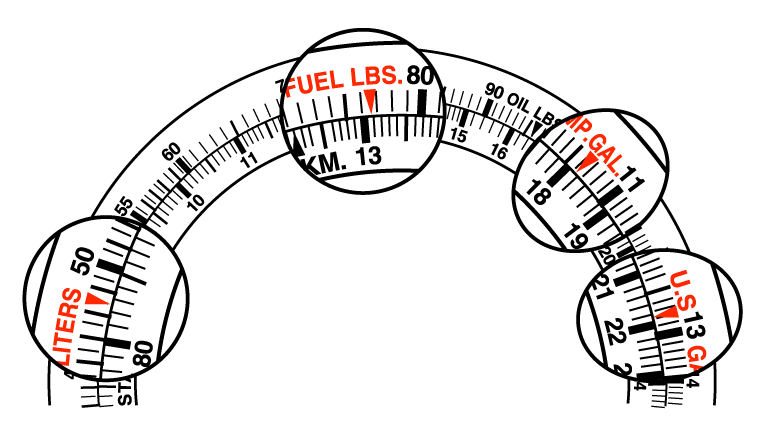You can convert fuel between Pounds (lbs), U.S. Gallons, Imperial Gallons or Liters.

Q :
How much is 13.1 lbs of fuel if it is converted into U.S. Gallons, Imperial Gallons and Liters respectively?
(1 FUEL LBS. is 0.167 U.S.GAL. / 0.139 IMP.GAL. /0.632 Liters)

A :
Adjust the “▼” of FUEL LBS. of the outer scale until it points to the digit 13.1, which you want to convert, of the inner scale.
Read the digit corresponding to the “▼” of U.S.GAL. of the outer scale. Adding a unit and you will get the answer which is 2.18 U.S.GAL.
Similarly, read the digit corresponding to the “▼” of IMP.GAL., LITERS, you can get the answers, which are 1.82 IMP.GAL. and 8.28 Liters.

### [How to convert weight between the different units of measurement]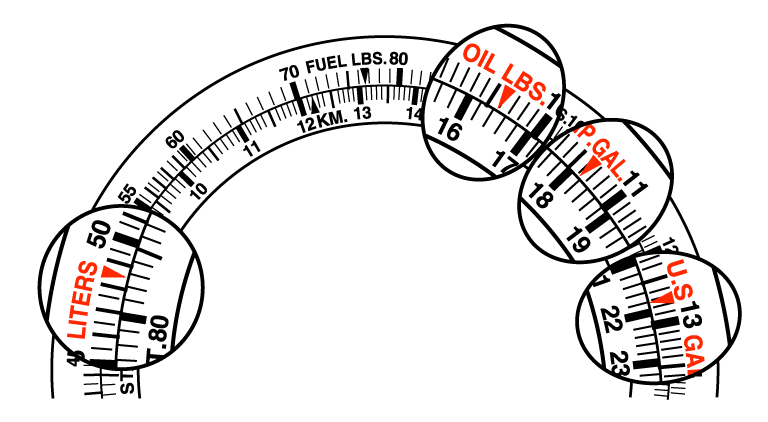You can convert between Oil in Pounds to U.S. Gallons, Imperial Gallons or Liters.

Q :
How much is 16.4 lbs of oil converted into U.S. Gallons, Imperial Gallons and Liters respectively?
(1 OIL LBS. is 0.133 U.S.GAL., 0.111 IMP.GAL. and 0.503 Liters)

A :
Adjust the “▼” of OIL LBS. of the outer scale until it points to the digit 16.4, which you want to convert, of the inner scale.
Read the digit corresponding to the “▼” of U.S.GAL. of the outer scale. Adding a unit and you will get the answer which is 2.18 U.S.GAL.
Similarly, read the digit corresponding to the “▼” of IMP. GAL., LITERS, you can get the answers, which are 1.82 IMP.GAL. and 8.25 Liters.

### [How to convert distance between the different units of measurement]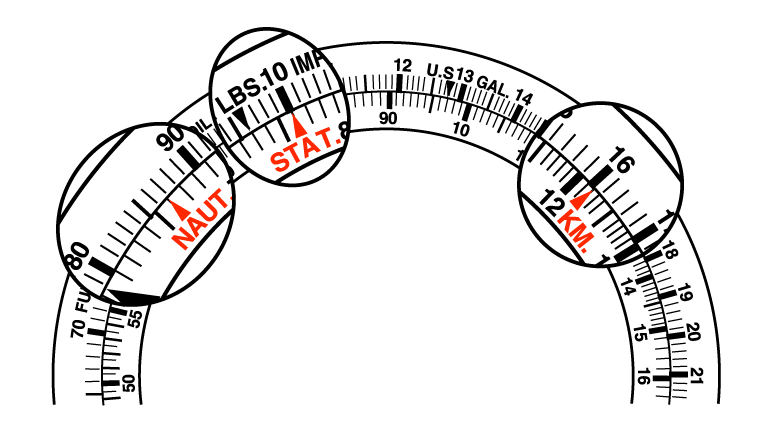You can convert between Kilometers, Nautical Miles and Statute miles.

Q :
How many Kilometers and Nautical Miles are equivalent to 1 Statute Mile?

A :
Adjust the “▼” of 10 of the outer scale until it points to the “▲” of STAT. of the inner scale.
Result: Read 16 of the scale corresponding to the “▲” of KM. of the inner scale. Move the decimal point once, and you will get the answer which is 1.6km.
Similarly, you can get the answer 86.6 NAUT (0.866 Nautical Miles) corresponding to the “▲” of NAUT.

### [How to convert fuel between different units of measurement]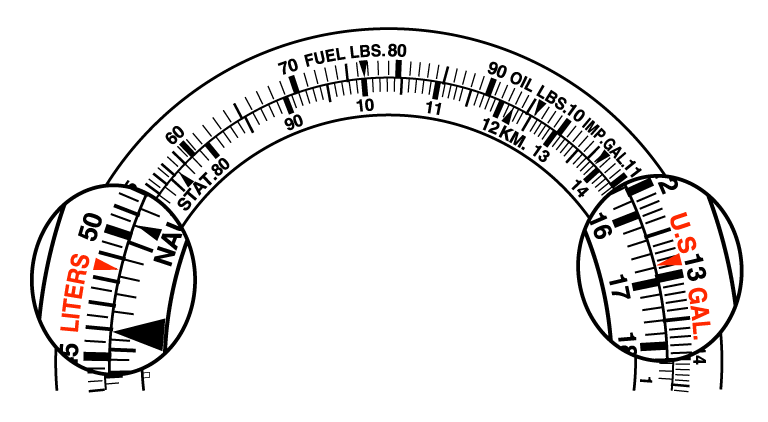You can convert between Liters, U.S. Gallons or Imperial Gallons.

Q :
How many Liters are equivalent to 16.8 U.S. Gallons?

A :
Align the “▼” of U.S.GAL. of the outer scale with the digit 16.8, which you want to convert, of the inner scale.
Result: The converted value (about 63.5) corresponding to the “▼” of liter of the outer scale can be determined.
(1 U.S.GAL. = 3.78541 Liters)

## How to use the motor sports function

### [Calculation of time needed to reach a set distance]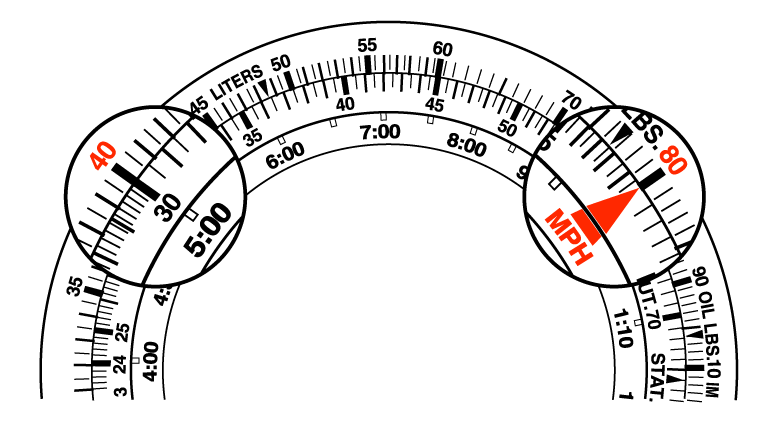Q :
How long does it take for a vehicle travelling at a speed of 80km to travel 400km?

A :
Align 80 of the outer scale with the SPEED INDEX “▲” of the inner scale.
Result: 5 Hours (5:00) of the inner scale corresponds to 40 of the outer scale.

### [Calculation of speed]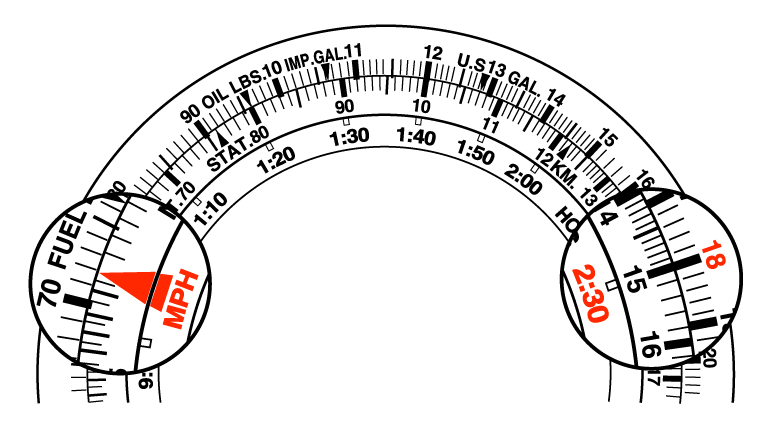Q :
What is the speed of a vehicle travelling a distance of 180km for period 2 hours and 30 minutes?

A :
Align 18 of the outer scale with 2:30 of the inner scale.
Result: 72km can be determined by comparing the SPEED INDEX “▲” of the inner scale and corresponding outer scale indications.

### [Calculation of driving distance]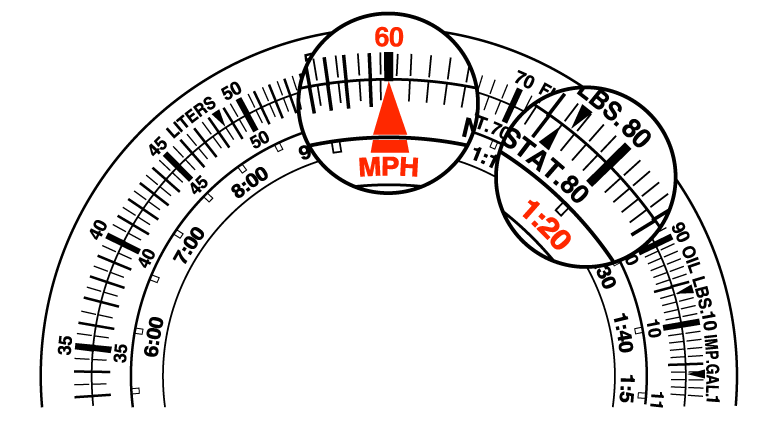Q :
Supposing that the speed is 60km/hour, what distance will a vehicle travel in 1 hour and 20 minutes?

A :
Align 60 of the outer scale with the SPEED INDEX “▲” of the inner scale.
Result: 80km corresponding to 1:20 of the inner scale can be figured out.

### [Calculation of fuel consumption rate (consumed per hour)]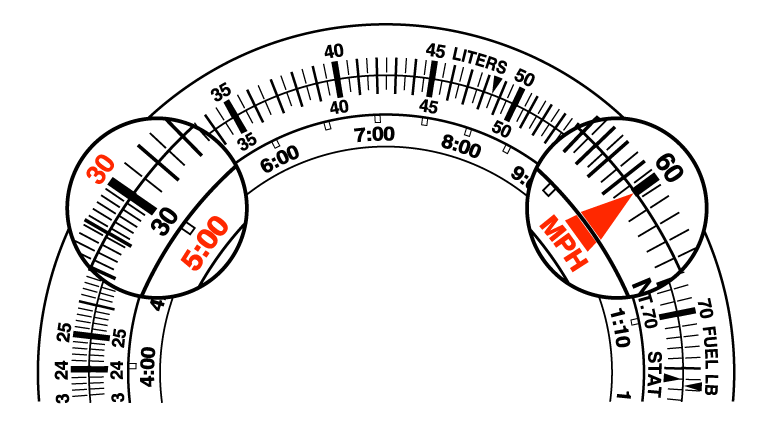Q :
If the drive time is 5 hours and 30 liters fuel are consumed, what is the fuel consumption rate (liters/hour)?

A :
Align 30 of the outer scale with 5:00 of the inner scale.
Result: 6 Liters per hour - 60 is indicated on the SPEED INDEX “▲” corresponding to the outer scale.

### [Calculation of fuel consumption]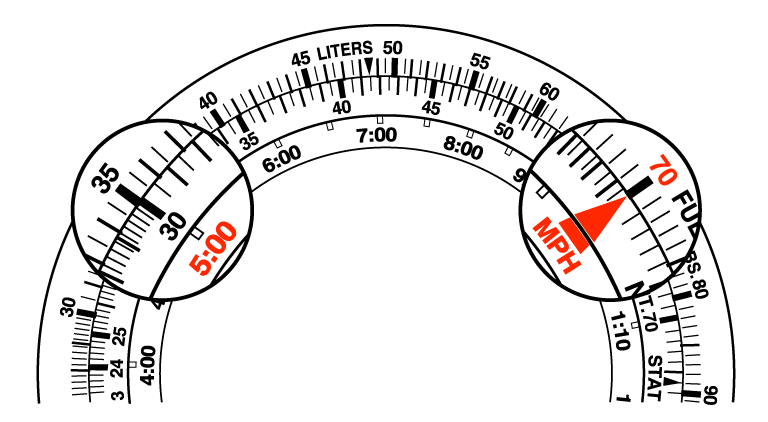Q :
How much fuel is needed for a vehicle to run 5 hours if the fuel consumption rate of that vehicle is 7 liters per hour?

A :
Align 70 of the outer scale with the “▲” of SPEED INDEX of the inner scale.
Result: 35 liters - 35 corresponds to 5.

### [Calculation of drive time]Q :
How many hours can a vehicle whose fuel consumption rate is 8 liters/hour run using 40 liters of fuel?

A :
Align 80 of the outer scale with the SPEED INDEX “▲” of the inner scale.
Result: 5 Hours - 5:00 on the inner scale corresponds to 40 of the outer scale.

## How to use the aviation function

### [Calculation of time needed to travel a set distance]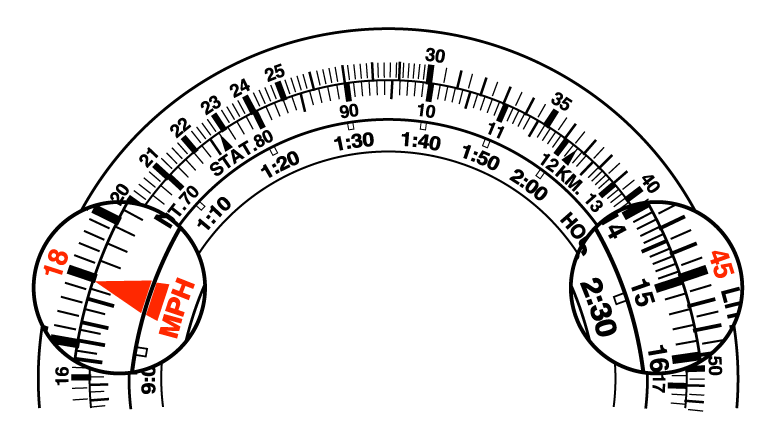Q :
If the speed of an aircraft is 180 knots, how much time does it take the aircraft to fly 450 nautical miles?

A :
Align 18 of the outer scale with the SPEED INDEX “▲” of the inner scale.
Result: 2 hours and 30 minutes - 2:30 of the inner scale corresponds to 45 of the outer scale.

### [Calculation of flight distance]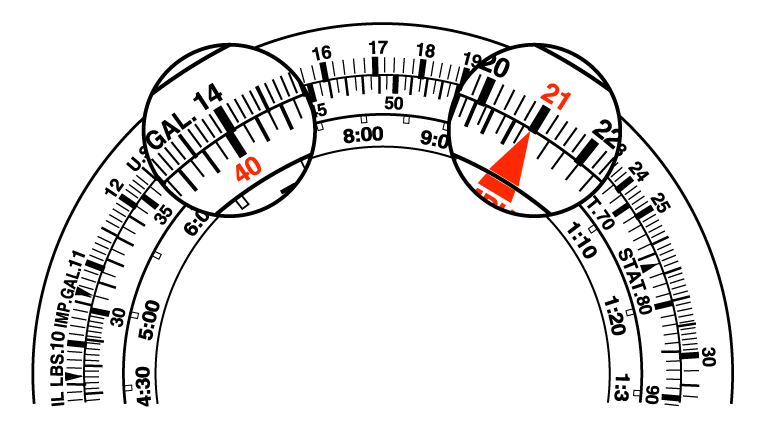Q :
If the speed is 210 knots and the flight time is 40 minutes, how many nautical miles will an aircraft travel?

A :
Align 21 of the outer scale with the SPEED INDEX “▲” of the inner scale.
Result: 140 Nautical Miles – 14 on the outer scale corresponds to 40 of the inner scale.

### [Calculation of fuel consumption rate (consumption per hour)]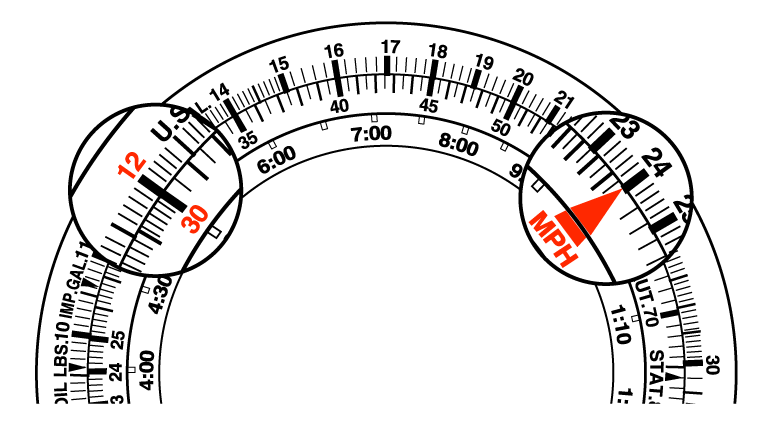Q :
If the flight time is 30 minutes and 120 gallons fuel is consumed, what is the fuel consumption rate?

A :
Align 12 of the outer scale with 30 of the inner scale. Result: 240 gallons per hour - 24 corresponding to the SPEED INDEX “▲”.

### [Calculation of fuel consumption]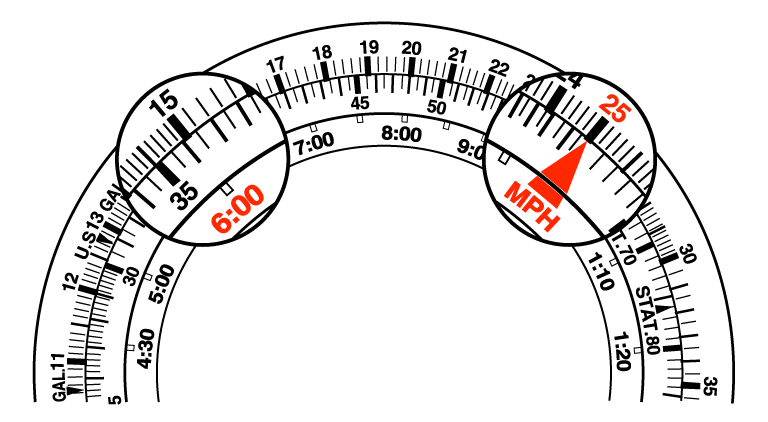Q :
If the fuel consumption rate of an aircraft is 250 gallons/hour, how many gallons of fuel are needed to fly 6 hours?

A :
Align 25 of the outer scale with the “▲” of SPEED INDEX of the inner scale.
Result: 1,500 gallons - 15 corresponds to 6:00.

### [Calculation of the flight time]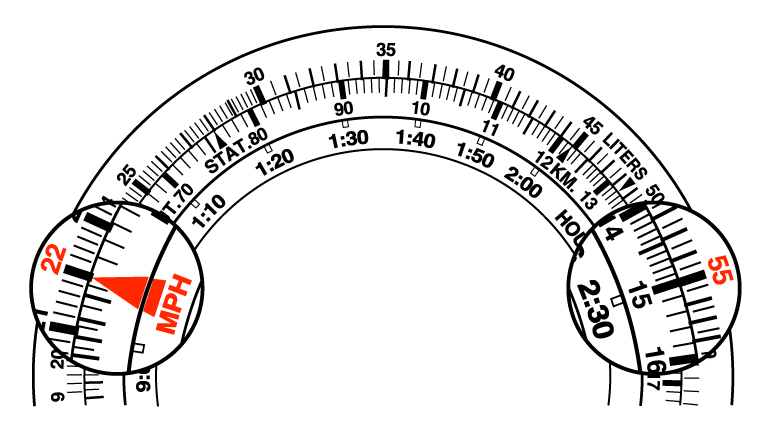Q :
If an aircraft consumes 220 gallons per hour, how many hours can it fly when consuming 550 gallons fuel?

A :
Align 22 of the outer scale with the “▲” of SPEED INDEX of the inner scale.
Result: Two hours and thirty minutes - 2:30 corresponds to 55 of the outer scale.